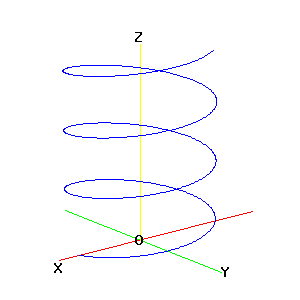Egwald Mathematics: Space Curves

by

Elmer G. Wiens

Egwald's popular web pages are provided without cost to users.
Please show your support by joining Egwald Web Services as a Facebook Fan:Follow Elmer Wiens on Twitter:Space Curves

Suppose that the location of the point P(x,y,z) in three dimensional space is determined as a function of time t. Specifically, suppose that:

x = x(t),   y = y(t),   z = z(t)

Thus, each component of P is a separate function of time. Then as t varies, the locus of P is a curve in three dimensional space.

For example, the following equations will trace a circular helix.

x = a * cos(w * t),   y = b * sin(w * t),   z = c * t

 In the example to the right, a = 1.5, b = 1.5, c = .1, w = pi/6, 0 < t < 40 T = the tangent to the curve at P with t = 16 N = the normal to the curve at P B is perpendicular to T and N B = T X N, where X denotes cross product The system of mutually orthogonal vectors, T, N, and B, are used extensively in analyzing space curves in general.Let the vector from the origin to the point P be R. Let s be the arc length along the space curve from P to another point P0. Then

R = i * x(t) + j * y(t) + k * z(t)

where i, j, and k are the unit vectors along the x, y, and z axis, respectively.

For a circular helix:

R = i * a * cos(w * t) + j * b * sin(w * t) + k * c * t

To obtain the unit tangent vector, T, to the space curve at P, differentiate R with respect to s:

T = dR / ds = { i*(-a*w*sin(w*t) + j*(b*w*cos(w*t)) + k*c } dt / ds

For T to be a unit vector requires that the magnitude of T be 1. So,

dt / ds = 1 / sqrt[w2 * (a2 * sin2(w*t) + b2 * cos2(w*t)) + c2] = kap.

To obtain the unit normal vector, N, to the space curve at P, differentiate T with respect to s, dT / ds = kappa * N, with |N| = 1.

dT / ds = (dT/dt)/(dt/ds) = kap * { i*(a*w2*cos(w*t) + j*(b*w2(sin(w*t))} * dt / ds

|dT/ds| = kappa = kap2 * w2 * sqrt[ a2*cos2(w*t) + b2 * sin2(w*t)]

Kappa is the curvature of the space curve at P.

Notes:
1. The velocity vector, V(t) = dR / dt, in the direction of T, is given by

V = dR / dt = i*(-a*w*sin(w*t) + j*(b*w*cos(w*t)) + k*c.

2. The acceleration vector, A(t) = dV / dt is given by

A = dT / dt = i*(a*w2*cos(w*t) + j*(b*w2(sin(w*t)).

3. When a = b, matters simplify because cos2(w*t) + sin2(w*t) = 1. Then

kappa = a * w2 / (a2 * w2 + b2)

 Space Curves - Helix Reset value of a [.5, 2.5]x = a * cos(w*t) 2 Reset value of b [.5, 2.5]y = b * cos(w*t) 1.5 Reset value of c [.05, .5]z = c * t 0.1Copyright © Elmer G. Wiens:   EgwaldWeb ServicesAll Rights Reserved.    Inquiries